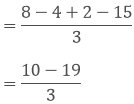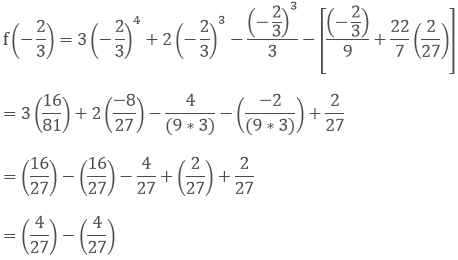×#### Thank you for registering.

One of our academic counsellors will contact you within 1 working day.

Click to Chat

1800-1023-196

+91-120-4616500

CART 0

• 0

MY CART (5)

Use Coupon: CART20 and get 20% off on all online Study Material

ITEM
DETAILS
MRP
DISCOUNT
FINAL PRICE
Total Price: Rs.

There are no items in this cart.
Continue Shopping• Complete JEE Main/Advanced Course and Test Series
• OFFERED PRICE: Rs. 15,900
• View Details

```Chapter 6: Factorization of Polynomials Exercise – 6.3

Question: 1

Using the remainder theorem, find the remainder when f(x) is divided by g(x) and verify the by actual division

f(x) = x3 + 4x2 − 3x + 10, g(x) = x + 4

Solution:

Here,  f(x) = x3 + 4x2 − 3x + 10

g(x) = x + 4

from, the remainder theorem when f(x) is divided by g(x) = x - (- 4)  the remainder will be equal to f(- 4)

Let, g(x) = 0

⟹ x + 4 = 0

⟹ x = - 4

Substitute the value of x in f(x)

f(-4) = (- 4)3 + 4(- 4)2 - 3(- 4) + 10

= - 64 + (4*16) + 12 + 10

= - 64 + 64 + 12 + 10

= 12 + 10

= 22

Therefore, the remainder is 22

Question: 2

Using the remainder theorem, find the remainder when f(x) is divided by g(x) and verify the by actual division

f(x) = 4x4 - 3x3 - 2x2 + x - 7, g(x) = x - 1

Solution:

Here, f(x) = 4x4 - 3x3 - 2x2 + x -7

g(x) = x - 1

from, the remainder theorem when f(x) is divided by g(x) = x - (-1) the remainder will be equal to f(1)

Let, g(x) = 0

⟹ x - 1 = 0

⟹ x = 1

Substitute the value of x in f(x)

f(1) = 4(1)4 - 3(1)3 - 2(1)2 + 1 - 7

= 4 - 3 - 2 + 1 - 7

= 5 - 12

= -7

Therefore, the remainder is 7

Question: 3

Using the remainder theorem, find the remainder when f(x) is divided by g(x) and verify the by actual division

f(x) = 2x4 - 6x3 + 2x2 - x + 2, g(x) = x + 2

Solution:

Here, f(x) = 2x4 - 6x3 + 2x2 - x + 2

g(x) = x + 2

from, the remainder theorem when f(x) is divided by g(x) = x - (-2) the remainder will be equal to f(-2)

Let, g(x) = 0

⟹ x + 2 = 0

⟹ x = - 2

Substitute the value of x in f(x)

f(-2) = 2(−2)4 - 6(-2)3 + 2(-2)2 - (-2) + 2

= (2 * 16) - (6 * (-8)) + (2 * 4) + 2 + 2

= 32 + 48 + 8 + 2 + 2

= 92

Therefore, the remainder is 92

Question: 4

Using the remainder theorem, find the remainder when f(x) is divided by g(x) and verify the by actual division

f(x) = 4x3 - 12x2 + 14x - 3, g(x) = 2x - 1

Solution:

Here, f(x) = 4x3 - 12x2 + 14x - 3

g(x) = 2x - 1

from, the remainder theorem when f(x) is divided by g(x) = 2(x - 1/2), the remainder is equal to f(1/2)

Let, g(x) = 0

⟹ 2x - 1 = 0

⟹ 2x = 1

⟹ x = 1/2

Substitute the value of x in f(x)

f(1/2) = 4(1/2)3 - 12(1/2)2 + 14(1/2) - 3

= 4(1/8) - 12(1/4) + 4(1/2) - 3

= (1/2) - 3 + 7 - 3

= (1/2) + 1

Taking L.C.M= (3/2)

Therefore, the remainder is (3/2)

Question: 5

Using the remainder theorem, find the remainder when f(x) is divided by g(x) and verify the by actual division

f(x) = x3 − 6x2 + 2x − 4, g(x) = 1 - 2x

Solution:

Here, f(x) = x3 - 6x2 + 2x - 4

g(x) = 1 - 2x

from, the remainder theorem when f(x) is divided by g(x) = -2(x - 1/2), the remainder is equal to f(1/2)

Let, g(x) = 0

⟹ 1 - 2x = 0

⟹ -2x = -1

⟹ 2x = 1

⟹ x = 1/2

Substitute the value of x in f(x)

f(1/2) = (1/2)³ - 6(1/2)² + 2(1/2) - 4

= 1/8 - 8(1/4) + 2(1/2) - 4

= 1/8 - (1/2) + 1 - 4

= 1/8 - (1/2) - 3

Taking L.C.MTherefore, the remainder is (-35)/8

Question: 6

Using the remainder theorem, find the remainder when f(x) is divided by g(x) and verify the by actual division

f(x) = x4 − 3x2 + 4, g(x) = x - 2

Solution:

Here, f(x) = x4 - 3x2 + 4

g(x) = x - 2

from, the remainder theorem when f(x) is divided by g(x) = x - 2 the remainder will be equal to f(2)

Let, g(x) = 0

⟹ x - 2 = 0

⟹ x = 2

Substitute the value of x in f(x)

f(2) = 24 - 3(2)2 + 4

= 16 - (3* 4) + 4

= 16 - 12 + 4

= 20 - 12

= 8

Therefore, the remainder is 8

Question: 7

Using the remainder theorem, find the remainder when f(x) is divided by g(x) and verify the by actual division

f(x) = 9x3 − 3x2 + x − 5, g(x) = x − 2/3

Solution:

Here, f(x) = 9x3 − 3x2 + x − 5

g(x) = x − 2/3

from, the remainder theorem when f(x) is divided by g(x) = x - 2/3 the remainder will be equal to f(2/3)

substitute the value of x in f(x)

f(2/3) = 9(2/3) - 3(2/3)² + (2/3) - 5

= 9(8/27) − 3(4/9) + 2/3 − 5

= (8/3) − (4/3) + 2/3 − 5= - 9/3

= - 3

Therefore, the remainder is - 3

Question: 8

Using the remainder theorem, find the remainder when f(x) is divided by g(x) and verify the by actual division

f(x) = 3x4 + 2x3 − x3/3 − x/9 + 2/27, g(x) = x + 2/3

Solution:

Here, f(x) = 3x4 + 2x3 − x3/3 − x/9 + 2/27

g(x) = x + 2/3

from remainder theorem when f(x) is divided by g(x) = x - (-2/3), the remainder is equal to f(- 2/3)

substitute the value of x in f(x)= 0

Therefore, the remainder is 0

Question: 9

If the polynomial 2x3 + ax2 + 3x − 5 and x3 + x2 − 4x + a leave the same remainder when divided by x - 2, Find the value of a

Solution:

Given, the polymials are

f(x) = 2x3 + ax2 + 3x − 5

p(x) = x3 + x2 − 4x + a

The remainders are f(2) and p(2) when f(x) and p(x) are divided by x - 2

We know that,

f(2) = p(2)  (given in problem)

we need to calculate f(2) and p(2)

for, f(2)

substitute (x = 2) in f(x)

f(2) = 2(2)3 + a(2)2 + 3(2) − 5

= (2 * 8) + 4a + 6 - 5

= 16 + 4a + 1

= 4a + 17 .... 1

for, p(2)

substitute (x = 2) in p(x)

p(2) = 23 + 22 - 4(2) + a

= 8 + 4 - 8 + a

= 4 + a .... 2

Since, f(2) = p(2)

Equate eqn 1 and 2

⟹ 4a + 17 = 4 + a

⟹ 4a - a = 4 - 17

⟹ 3a = -13

⟹ a = -13/3

The value of a = −13/3

Question: 10

If polynomials ax3 + 3x2 − 3 and 2x3 − 5x + a when divided by (x - 4) leave the remainders as R1 and R2 respectively. Find the values of a in each of the following cases, if

1. R1 = R2

2. R1 + R2 = 0

3. 2R1 − R2 = 0

Solution:

Here, the polynomials are

f(x) = ax3 + 3x2 - 3

p(x) = 2x3 - 5x + a

Let,

R1 is the remainder when f(x) is divided by x - 4

⟹ R1 = f(4)

⟹ R1 = a(4)3 + 3(4)2 − 3

= 64a + 48 - 3

= 64a + 45 .... 1

Now, let

R2 is the remainder when p(x) is divided by x - 4

⟹ R2 = p(4)

⟹ R2 = 2(4)3 - 5(4) + a

= 128 - 20 + a

= 108 + a   .... 2

1. Given, R1 = R2

⟹ 64a + 45 = 108 + a

⟹ 63a = 63

⟹ a = 1

2. Given, R1 + R2 = 0

⟹ 64a + 45 + 108 + a = 0

⟹ 65a + 153 = 0

⟹ a = −153/65

3. Given, 2R1 − R2 = 0

⟹ 2(64a + 45) - 108 - a = 0

⟹ 128a + 90 - 108 - a = 0

⟹ 127a - 18 = 0

⟹ a = 18/127

Question: 11

If the polynomials ax3 + 3x2 − 13 and 2x3 − 5x + a when divided by (x - 2) leave the same remainder, Find the value of a

Solution:

Here, the polynomials are

f(x) =  ax3 + 3x2 − 13

p(x) = 2x3 − 5x + a

equate, x - 2 = 0

x = 2

substitute the value of x in f(x) and p(x)

f(2) = (2)3 + 3(2)2 − 13

= 8a + 12 - 13

= 8a - 1 ..... 1

p(2) = 2(2)3 - 5(2) + a

= 16 - 10 + a

= 6 + a .... 2

f(2) = p(2)

⟹ 8a - 1 = 6 + a

⟹ 8a - a = 6 + 1

⟹ 7a = 7

⟹ a = 1

The value of a = 1

Question: 12

Find the remainder when x3 + 3x3 + 3x + 1 is divided by,

1. x + 1

2. x - 1/2

3. x

4. x + π

5. 5 + 2x

Solution:

Here, f(x) = x3 + 3x2 + 3x + 1

by remainder theorem

1 ⟹ x + 1 = 0

⟹ x = -1

substitute the value of x in f(x)

f(-1) = (−1)3 + 3(−1)2 + 3(−1) + 1

= -1 + 3 - 3 + 1

= 0

2. x - 1/2

Here, f(x) = x3 + 3x2 + 3x + 1

By remainder theorem

⟹ x - 1/2 = 0

⟹ x = 1/2

substitute the value of x in f(x)

f(1/2) = (1/2)³ + 3(1/2)² + 3(1/2) + 1

= (1/2)3 + 3(1/2)2 + 3(1/2) + 1

= 1/8 + 3/4 + 3/2 + 1= 27/8

3. x

Here, f(x) = x3 + 3x2 + 3x + 1

by remainder theorem

⟹ x = 0

substitute the value of x in f(x)

f(0) = 03 + 3(0)2 + 3(0) + 1

= 0 + 0 + 0 + 1

= 1

4. x + π

Here, f(x) = x3 + 3x2 + 3x + 1

by remainder theorem

⟹ x + π = 0

⟹ x = - π

Substitute the value of x in f(x)

f(- π) = (- π)3 + 3(-π)2 + 3(-π) + 1

= -(π)3 + 3(π)2 − 3(π) + 1

5. 5 + 2x

Here, f(x) = x3 + 3x2 + 3x + 1

by remainder theorem

5 + 2x = 0

2x = -5

x = -5/2

substitute the value of x in f(x)

f(-5/2) = (-5/2)³ + 3(-5/2)² + 3(-5/2) + 1

= −125/8 + 3(25/4) + 3(-5/2) + 1

= −125/8 + 75/4 - 15/2 + 1

Taking L.C.M= −27/8
```### Course Features

• 728 Video Lectures
• Revision Notes
• Previous Year Papers
• Mind Map
• Study Planner
• NCERT Solutions
• Discussion Forum
• Test paper with Video Solution